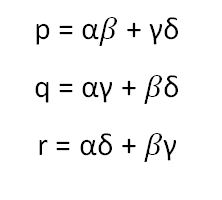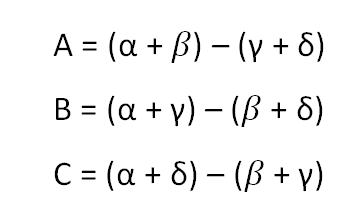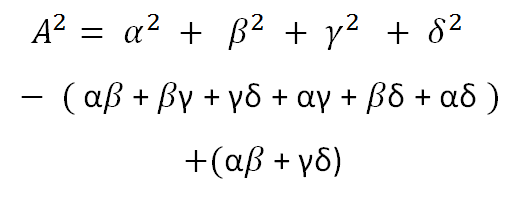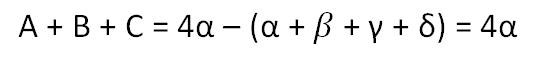## Tuesday, July 17, 2012

### Solving the Fourth Degree: Part II

When we left off, we had just reached the halfway point in our solution of the quartic equation. What we had done was to show the steps necessary to write explicit expressions p, q, and r for these three peculiar functions of alpha, beta, gamma and delta:The p, q and r turned out to be the roots of a cubic with rational coefficients that we can easily calculate. Our alphas and betas etc. are of course the roots of our quartic. Now let's see how we can extract them individually from our p's and q's etc.

The next step is to consider all possible combinations of the alphas and betas etc. with alternating sign:There are just three more possible combinations, but they amount to what we already have with opposite signs. What I want to show you now is that our A, B, and C are actually the square root of numbers that we have access to via our p's and q's etc! You can verify this by squaring A for example:Look what we have. The first line in alpha-squared etc. is symmetric in alpha-beta-gamma-delta, so it's just a rational number. The second line in alpha-beta etc. is also symmetric...in fact, it's just the coefficient of the linear term in the quartic equation. So it's also a rational number. The third line is our p, the number we said was the solution of a cubic equation for which we know the coefficients. So in other words, our A is just the square root of (p + b), where b is a rational number.

It's obvious that our B and C are similarly just the square roots of similar functions which we can write in radicals. So we can write A, B, and C explicitly...at least up to the ambiguity of a minus sign. But look what we get for the sum of A, B, and C:By adding up A, B, and C we isolate alpha! Remember it's easy to make the second-from-leading coefficient in any polynomial equal to zero by a simple linear shift, which knocks out the bracketed term in the above expression. (It's called "completing the square" when we do it on a quadratic equation.) We've shown an explicit expression which solves the quartic equation.

What about beta, gamma and delta? Remember there was an ambiguity when we took square roots. We might have chosen alternate signs in each case. The different choices for the sign of the square root lead to sums of A, B and C which are variously beta, gamma and delta depending on our choices.

And that's how you solve the fourth-degree equation.

#### 1 comment:Anonymous said...

Nice explanation. It is just that A squared was not calculated exactly. Zero sum of the roots could be used in that calculation, too.